Applying Hough Transform for detecting lines in imagesDownload Article, Applet and Source (170 kb)View applet online now!

Introduction

The Hough transform is an algorithm to detect objects in an image. Originally, it was invented to find lines in images, later enhanced to detect circles and meanwhile there are a number of modified & improved algorithms to detect various features in images analysis.

This article is focused on finding lines in images by applying the hough transform algorithm. Thereby I will concentrate an the practical side: on the one side the details of the process are explained in a way to fully understand what happens during the hough transform process, on the other side I will also focus on my implementation.
I have come out to implement the algorithm in succesive steps. As source an arbitrary image can be loaded, then following steps are applied one after another to detect the lines:

Please note that I have created my implementation step by step along with theoretically dealing with the algorithm - it's not fast, the result is not optimal but at least it works. Additionally all immediate steps are visualized which may turn out to be the crucial point of this tutorial. Nevertheless, I hope you like it so let's start.

I. Converting the colored image to grayscale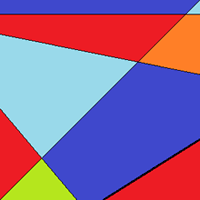==>This step is actually no big deal. One possibility is to iterate over each color pixel and calculate the luminance value using following formula:

luminance = 0.3 * r + 0.59 * g + 0.11 * b (where r,g and b are the color components of a RGB color value).

[Interesting side note: Of course one could also use the (more intuitive) conversion luminance = 1/3 * r + 1/3 * g + 1/3 * b, but this gives worse result. The reason is that the human eye is not equally sensitive to the three fundamental colors and the factors 0.3, 0.59 and 0.11 do much better fit to the light sensitiveness of the receptors of the human eye.]

Another solution is to use framework function if available; here is what I use in Java:

 BufferedImage grayImage = new BufferedImage(colorImage.getWidth(), colorImage.getHeight(), BufferedImage.TYPE_BYTE_GRAY); Graphics g = grayImage.getGraphics(); g.drawImage(colorImage.getImage(), 0, 0, null); g.dispose();

II. Edge Detection==>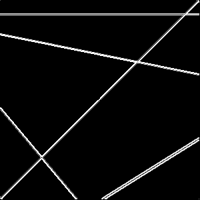In the next step the contours are extracted because the lines to be found can only lie on the outline. Therefore a gradient-based edge detection algoritm can be used like a Prewitt, Sobel or any other edge filter operator. For simplicity I have chosen to use a sobel filter.

The sobel operator is applied to each point in the image. At each point a horizontal and vertical filter is applied to detect changes in intensity of this point compared to its direct neighbour points. A high difference in intensity of side-by-side point implies then a part of an edge. On the other side, if a point and its neighbour points have the same grayscale value, the operator value will be zero which means no change in intensity and thus no possible edge.

The sobel operator uses two 3×3 kernels which are convolved with the original image to calculate approximations of the gradients. Operator Sx detects the changes in horizontal (x-) direction while Sy does the same for the y-direction and are defined as

 1 0 -1 Sx = 2 0 -2 1 0 -1
 1 2 -1 Sy = 0 0 0 -1 -2 -1

Example:

As an example assume below is a small extract of a grayscale image. The image point under investigation has the grayvalue of 80, the three neighbour to the left have larger grayscale values (thus are brighter), on ones on the right are darker. Now the Sx filter is applied to this portion:

 140 90 60 135 80 50 130 90 50

Then the Sx operator would calculate the following value:
Gx = 140 * 1 + 90 * 0 + 60 * (-1) + 135 * 2 + 80 * 0 + 50 * (-2) + 130 * 1 + 90 * 0 + 50 * (-1) = 330.
Afterwards also the Sy operator is applied to the point which however results in a much smaller value because the change in intensity is far less strict in y-direction than in x-direction:
Gy = 140 * 1 + 90 * 2 + 60 * 1 + 135 * 0 + 80 * 0 + 50 * 0 + 130 * (-1) + 90 * (-2) + 50 * (-1) = 20.

Note that such edge value might also be negative depending on the direction of change of intensity - from dark to bright or from bright to dark. The absolute sobel edge value of a point is calculated by: G = sqrt(Gx2 + Gy2).
In case the edge value should be plotted it is necessary to clip it into a valid range, e.g. [0, 255].

III. Thresholded Edge Detection==>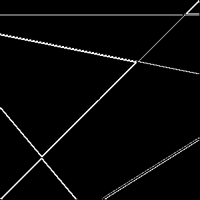After having calculated an edge value for each point, the decision must be performed if a point really belongs to an edge or not. The sobel operator provides a continuous range of edge values G but e.g. should a point with edge value 100 should really be considered as part of an edge or not?
Therefore a threshold T is applied to each point classifying it as edge or not:

 for each image point p {   if (G >= T)     p is part of an edge;   else     p is not part of an edge }

Obviously this is quite simple, but the tough part here is the determination of a good threshold. I have chosen the lame way in the test applet by letting the user configure the threshold value via a slider. A more sophisticated way would be to calculate the treshold depending on the maximum edge values and general intensity of the image (not handled here, sorry folks).

IV. Hough Transform==>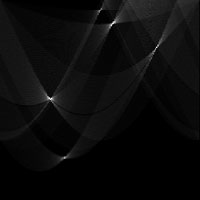Ok, now we come to the main step: Building the hough space from the thresholded edge image.
First some theory: The main idea is to transfrom the image space into another two-dimensional respresentation (the hough space) where lines are indicated by points. So all collinear points (these are points lying on the same line) are represented by the same coordinate in hough space.
Because a line is normally defined by an equation of form y = mx + c (where m is the slope and c the y-axis intercept), one could also describe image points (x,y) by its paremeters m and c. In other words, a line could be defined in a paremeter space spanned by parameters m and c.
However, this turns out not to be the best choice because the slope might also increase to infinity for lines parallel to the y-axis which means not all lines can be transformed into a finite parameter space.

A better approach is to use the normal form where each line is represented by a distance r (the distance between the closest point of the line to the origin) and angle Θ (angle between the normal vector from the clostest point of the line to the origin and the x-axis). Using this form, each line can be written as:

r = x * cos(Θ) + y * sin(Θ)

'Aehm.. ok' you are thinking now... magic! Don't worry, we are going to derive this equation. Look at following figure showing a line, an arbitrary point p on the line, the normal vector n of the line and our angle Θ, we are able to conclude following facts:r * n lies on our line (this a vector pointing to v in the figure right.)

Furthermore, for each point p we see that p - r * n is a vector that lies on the line (keep in mind this is vector subtraction)
==> this means that vector p - r * n is orthogonal to n
==> using the dot product (x) this can be written as (p - r * n) x n = 0
==> p x n - r * n x n = 0 and because n x n is 1 (becase length of n is 1 and the angle between n and n is 0° of course)
==> p x n - r * 1 = 0
==> p x n = r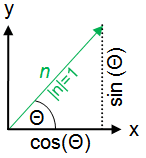Refering to the figure left, it's easy to see that the normal vector n can be written as a function of Θ.
cos(Θ) = x / |n|; but because the length of normal vector n is 1, the x-component of n is just cos(Θ).
The same applies for the y-component: sin(Θ) = y / |n|. With |n| = 1, this result in sin(Θ) = y.
So n = (cos(Θ), sin(Θ)).

Each point p (or better view it as vector p from origin to p) has the coordinate (x,y). With these facts follow:
p x n = r ==> (x, y) x (cos(Θ), sin(Θ)) = x * cos(Θ) + y * sin(Θ) = r. That's it!

Another point of view:Actually there is also another way to understand the equation x * cos(Θ) + y * sin(Θ) = r. Simple rearraging gives:
y = -cos(Θ)/sin(Θ) * x + r / sin(Θ).
This has the form of a normal line equation with slope m = -cos(Θ)/sin(Θ) and y-axis intercept c = r / sin(Θ). If you think about it, it makes absolutely sense! The normal vector has slope m' = sin(Θ)/cos(Θ). Because the actual line is orthogonal to the normal vector n and the relation of the slopes of two orthogonal lines is m1 = -1/m2, we get slope m = -1 / m' = 1 / sin(Θ)/cos(Θ) = -cos(Θ)/sin(Θ);
Looking at the figure to the right, it's also easy to see that sin(Θ) = r / c ==> c = r / sin(Θ);

The main fact to be learned form above formula is: Each line can be represented by one point in hough space.
Calculating the hough space is performed by iterating over each pixel in image space and check if it's an edge, thus if the corresponding pixel in the thresholded edge image is set. If so, the angle a and the distance r are calcuated and the tuple (a, r) is inserted into a two-dimensional array which is often called the voting matrix. [The angle a could be retrieved by the direction of the gradient from the edge detection step (angle a = tan-1(Gy/Gx). The other (lame) way is to calculate the tuple for each possible angle value]. The maxima in the voting matrix represent the actual lines in the image. The pseuo code (of the lame way) is something like this:

 for each image point p {   if (p is part of an edge)   {     for each possible angle     {       r = x * cos(angle) + y * sin(angle);       houghMatrix[angle][r]++;     }   } }The range of angle is sufficient to be [0°, 180°) because a line has no direction. I have chosen a step size of one full degree, so an array dimension of 180 for the angle, but this depends on the required precision. The range of r can be reckoned by the maximum distance of a line to the origin at a corner of the image, thus by sqrt(imagewidth2 + imageheight2). Because of sin() and cos() function (or better: depending on the direction of n), r might also be negative, so the range should be either [-r, r] or [0, 2r] when using an offset.
The figure on the right shows a visualization of a pseudo example: the blue line in the image would result in a maximum in hough space at angle = 45° and r = 4. The light gray sqaures around the actual maximum should hint to possible noise resulting from quanatization errors (e.g. angle quantization to a disrete range). That's why the hough space is also filtered in the next step.

V. Hough Space Filtering==>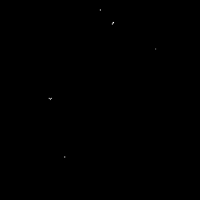Corresponding to the edge threshold algorithm of step III the hough space is threshold-filtered in order to remove the noise and to keep only the valid lines. Because the global maximum value of a point in hough space is not known a priori (in contrast to the maximum edge value), it must be estimated.
One approach is to take the image dimension into account and calculate the maximum line length - this can then be used to estimate the maximum hough space value.
Another way is to apply a relative threshold: Therefore the hough space is searched for the actual biggest value and then only the values are kept that exceed x percent of the global maximum (e.g >= 70% of the maxiumum). The latter procedure is used in the example applet.

VI. Converting lines to image space==>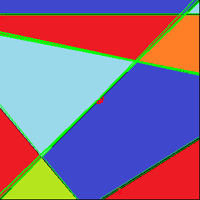Well, the actual hough transformation is finished at this point. However, it might be necessary to convert the lines back to image space, e.g. to overlay the original image with the detected lines as in the example applet. This procedure is not difficult - one possible algorithm consists of the following steps/facts:

1. Each point in hough space is given by angle a and distance r. Using these values, one single point p(x,y) of the line can be calculated by
px = r * cos(angle)
py = r * sin(angle).

2. The maximum length of a line is restricted by sqrt(imagewidth2 + imageheight2).

3. The point p, the angle a of the line and the maximum line length 'maxLength' can be used to calculate two other points of the line. The maximum length here ensures that both points to be calculated are lying outside of the actual image, resulting in the fact that if a line is drawn between these two points, the line goes from image border to image border in any case and is never cropped somewhere inside the image.

4. These two points p1 and p2 are calculated by:
p1_x = px + maxLength * cos(angle);
p1_y = py + maxLength * sin(angle);
p2_x = px - maxLength * cos(angle);
p2_y = py - maxLength * sin(angle);

5. Because p1 and p2 are lying outside the visible area of the image, it may be necessary the clip the final line between p1 and p2 against the 'borders' of the image. (Hm guess where you can find an awesome article about line clipping... maybe here? ;-)

The next logical step would be the cropping of the inifinite lines to the actual line segment which is not part of the article (at least not yet).

Conclusion

Hopefully you like this article and have learned something new and interesting.

Sunshine, December 2012

This site is part of Sunshine's Homepage